# On discontinuous value functions in time-optimal differential games

## Slide 1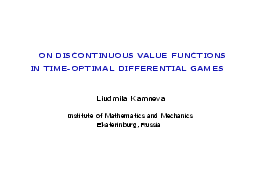My talk is devoted to study of discontinuous value functions in time-optimal differential games.

## Slide 2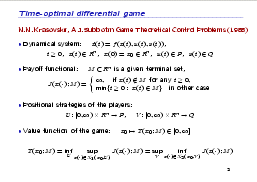The research is carried out in the context of the positional formalization of differential games developed by N.N.Krasovskii and A.I.Subbotin.

Let the motion of a dynamical system be described by the following equation. Here, x(t) is a phase state of the system; u(t) and v(t) are control realizations of the first (minimizing) and the second (maximizing) players with geometrical constraints; P and Q are compact sets. The payoff functional is the time of reaching a given closed terminal set M by a trajectory of the dynamical system. The first player minimizes the payoff, and the second player maximizes it from a given initial point.

The both players use feedback strategies. A feedback strategy of a player produces a family of constructive motions.

The value of the game is an optimal guaranteed result for the both players, and it is defined by the following equality. The value function assigns an optimal guaranteed result to each initial position of the game.

One of the approaches to solving differential games consists in finding the value function. The optimal feedback strategies of the players can be constructed on the basis of the value function.

## Slide 3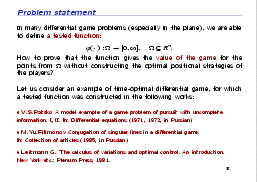In many differential game problems (especially in the plane), we are able to construct a tested function in some domain. The question is: how to proof that this function gives the value of the game for points from the domain without constructing optimal positional strategies of the players?

Firstly, I shall consider an example of a time-optimal differential game, for which a tested function was earlier constructed by other authors. Secondly, I shall present a new theorem on sufficient conditions for a function to coincide with the value function of the game.

## Slide 4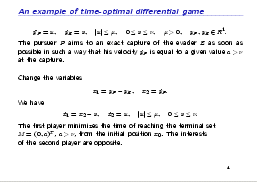Let's consider a pursuit-evasion game in a straight line with the following dynamics of the pursuer and the evader. The pursuer P aims to an exact capture of the evader E as soon as possible in such a way that his velocity is equal to a given value a > ν at the capture instant. After changing the variables, we obtain a time-optimal differential game in the plane with the following dynamics and the terminal set.

A tested function in the problem is constructed as a time of motion along a special field of trajectories.

## Slide 5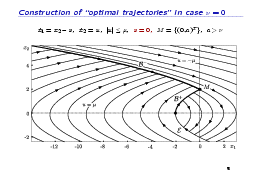For the case of one player (when there is a zero constraint on the control of the second player), the field of the trajectories is well known. We have two families of parabolas, which correspond to different extremal controls. The trajectories conjugate with each other on a special line. The tested function is discontinuous on a part of the line.

## Slide 6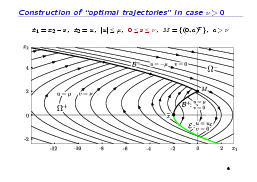For the general case of two players we have the same structure of parabolas, which corresponds to the following extremal controls, but the special line has more complicated description.

## Slide 7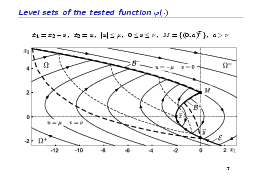Let us consider a time of motion along the trajectories of the field from an initial point x to the set M as a function of x.

We shall take a level set Ω of the function as the domain set for the tested function. Here, some intermediate level sets in Ω are shown.

## Slide 8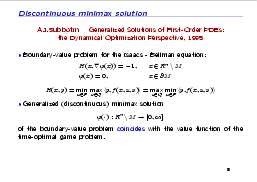It is well known that any time-optimal differential game corresponds to a boundary value problem for the Isaacs − Bellman equation. In the theory by Subbotin, the notion of discontinuous minimax solution to the problem is introduced. The fundamental result is that the value function of the game coincides with discontinuous minimax solution to the problem.

Let us note that there exists an analogous result in the theory of viscosity solutions.

To solve our problem, we need to check the conditions of a discontinuous minimax solution for the tested function. But the conditions are rather complicated to be directly used in concrete problems. Moreover, our tested function is not defined on the whole space Rn.

## Slide 9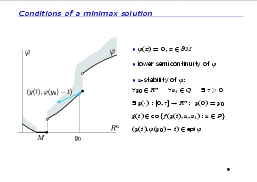The conditions of the minimax solution are the follows.

Firstly, there are the boundary condition, lower semi-continuity and u-stability of a function. The u-stability means weak invariance of the epigraph of the function with respect to the corresponding differential inclusion.

## Slide 10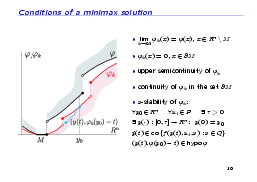Secondly, there exists a sequence of upper semi-continuous functions, which converges point-wisely to the given function. Each function of the sequence satisfies the boundary condition and v-stability property, and it is continuous on the boundary of the terminal set.

For a concrete tested function, difficulties arise when constructing the sequence of the functions mentioned above.

## Slide 11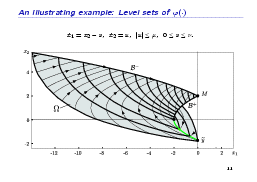A theorem on sufficient conditions for a function to coincide with the value function of the game was proved. I shall show the formulation of the theorem. The formulation will be illustrated by the example presented earlier.

## Slide 12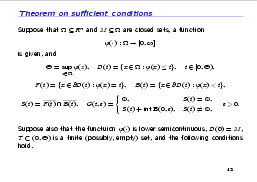Conditions of the theorem are imposed on each level set of the function except, may be, a finite number of the sets. On the border of a level set D(t), three subsets are defined: the set F(t) (a front) is the set where the tested function is equal to t, the set B(t) (a barrier) is the set where the tested function is less then t, and the set S(t) is the set of boundary points of the previous two sets.

## Slide 13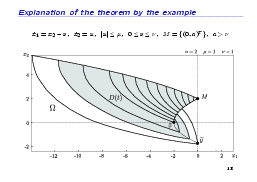Here, you can see a level set D(t) of the tested function, ...

## Slide 14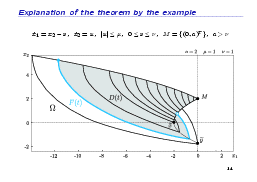... the front subset F(t) of the level set, ...

## Slide 15... the barrier subset B(t) of the level set, ...

## Slide 16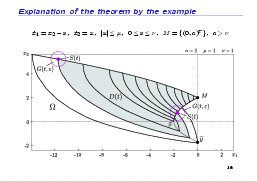... and the set S(t) of the boundary points.

## Slide 17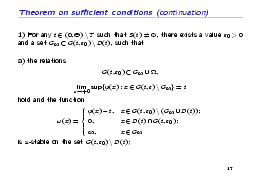The conditions of the theorem prescribe to check properties, which are analogous to the properties of a discontinuous minimax solution but in small neighbourhoods of the sets pointed out above. It means that there is a localization of minimax solution properties on small neighbourhoods. The localization simplifies the properties.

The properties are still complicated in a neighbourhood G(t, ε) of the set S(t).

One needs to check both the u-stability of the tested function and ...

## Slide 18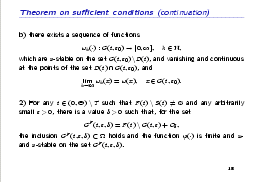... the existence of a sequence of v-stable functions with the corresponding boundary properties. This is the first condition of the theorem.

Here, the second condition related to the front points is written.

## Slide 19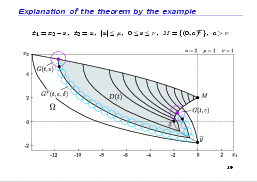In a neighbourhood of the front, the properties are reduced to checking u- and v-stability of the tested function.

## Slide 20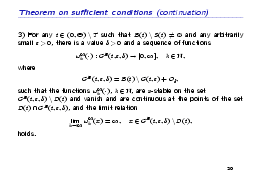Here, the third condition related to the barrier points is given.

## Slide 21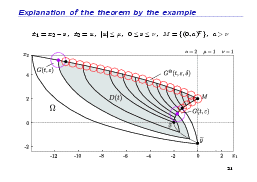In a neighbourhood of the barrier, the properties are reduced to checking an existence of a sequence of v-stable functions, which are degenerate in a certain sense and formally are not connected with the tested function.

## Slide 22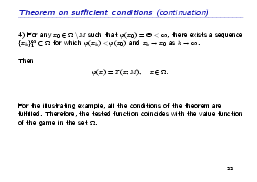The fourth condition has a technical character and is easy to verify.

If all the conditions are satisfied for each level set of the function except, may be, a finite number of the sets, then the tested function coincides with the value function of the game in the domain Ω.

For the illustrating example, all the conditions of the theorem are satisfied. Therefore, the tested function of the example coincides with the value function of the game.

## Slide 23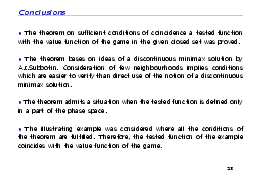1) The theorem on sufficient conditions of coincidence a tested function with the value function of the game in a given closed set was proved.

2) The theorem is based on ideas of a discontinuous minimax solution by A.I.Subbotin. Consideration of few neighbourhoods implies conditions, which are in some cases easier to verify than direct use of the notion of a discontinuous minimax solution.

3) The theorem admits a situation when the tested function is defined only on a part of the phase space.

4) The illustrating example was considered where all the conditions of the theorem are satisfied.